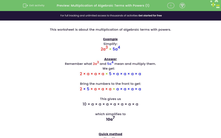# Multiply Algebraic Terms with Powers

In this worksheet, students will multiply algebraic terms.Key stage:  KS 3

Curriculum topic:   Algebra

Curriculum subtopic:   Simplify Algebraic Expressions to Maintain Equivalence

Popular topics:   Algebra worksheets

Difficulty level:#### Worksheet Overview

This activity is about the multiplication of algebraic terms with powers.

Example

Simplify:

2a3 × 5a4

Remember what 2a3 and 5a4 mean and multiply them.

We get:

2 × a × a × a × 5 × a × a × a × a

Bring the numbers to the front to get:

2 × 5 × a × a × a × a × a × a × a

This gives us

10 × a × a × a × a × a × a × a

which simplifies to

10a7

Quick method

Simplify:

2a3 × 5a4

Multiply the numbers (2 × 5 = 10).

Add the indices of identical letters (3 + 4 = 7).

Shall we have a go at some questions now?

### What is EdPlace?

We're your National Curriculum aligned online education content provider helping each child succeed in English, maths and science from year 1 to GCSE. With an EdPlace account you’ll be able to track and measure progress, helping each child achieve their best. We build confidence and attainment by personalising each child’s learning at a level that suits them.

Get started